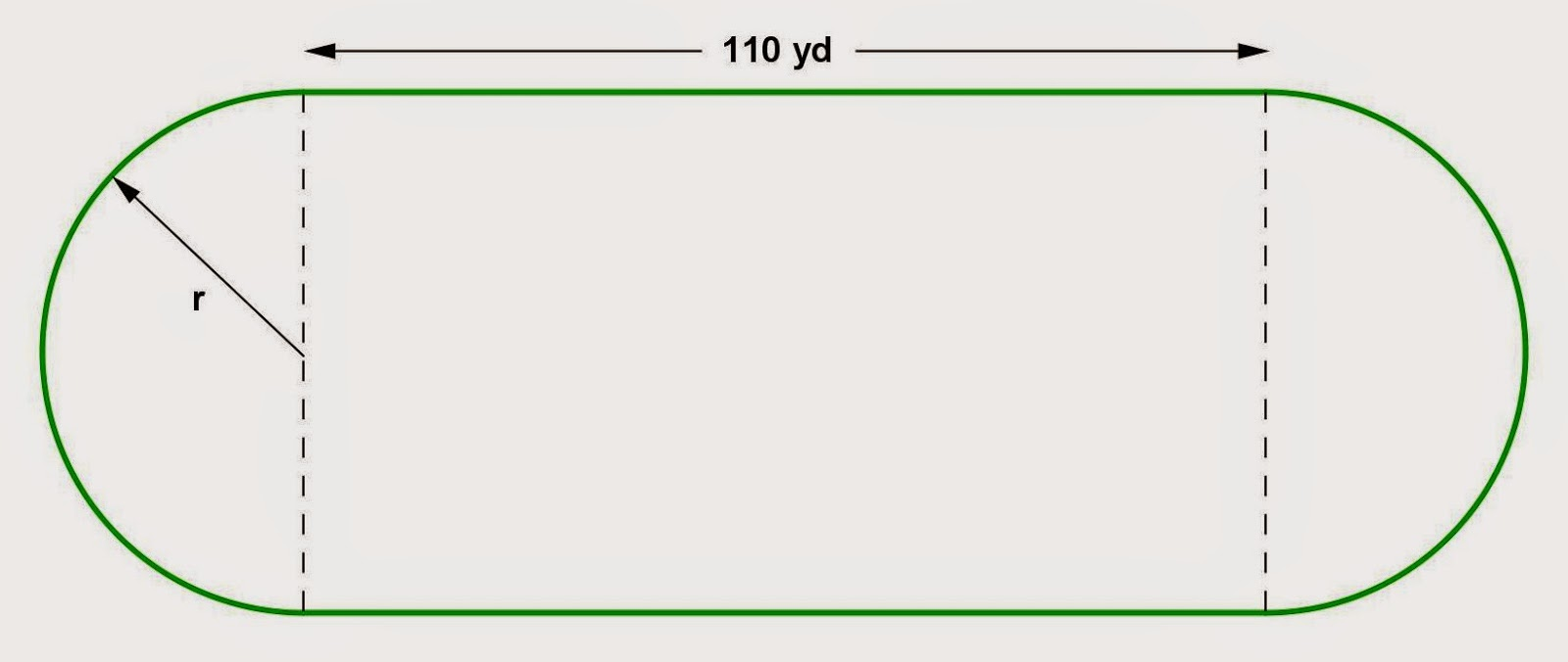## Wednesday, December 31, 2014

### Circle and Rectangle Problems, 2

Category: Plane Geometry

"Published in Vacaville, California, USA"

A running track has the shape shown in the figure, with straight sides and semicircular ends. If the length of the track is 440 yd and the two straight parts are each 110 yd long, what is the radius of the semicircular parts (to the nearest yard)?Photo by Math Principles in Everyday Life

Solution:

Consider the given figure abovePhoto by Math Principles in Everyday Life

Since the middle part of the running track is a rectangle, then the diameters of the two semicircular parts are equal. The width of a rectangle is equal to the diameter of two semicircles. If we combine the length of two semicircles, then it becomes a length or circumference of a circle. Hence, the length of a running track is

butTherefore, the radii of the semicircular parts of a running track are

## Tuesday, December 30, 2014

### Right Triangle Problems

Category: Plane Geometry

"Published in Vacaville, California, USA"

A city lot has the shape of a right triangle whose hypotenuse is 7 ft longer than one of the other sides. The perimeter of the lot is 392 ft. How long is each of the three sides of the lot?

Solution:

To illustrate the problem, it is better to draw the figure as followsPhoto by Math Principles in Everyday Life

Since there are two unknown variables for a right triangle, then we need to have two working equations in order to solve for the values of two variables.

The first working equation that we will use is Pythagorean Theorem because the figure is a right triangle. The first working equation is

Next, we need a second working equation which is the perimeter of a right triangle because it is given in the problem. The second working equation is

Substitute the value of b to the first working equation, we have

Since -1554 is not divisible by 4, then we have to use quadratic formula as follows

If you will choose a positive sign, then the value of a is

Hence, the value of b is

Since the value of b is negative, then we cannot accept the values of a and b in the first condition.

If you will choose a negative sign, then the value of a is

Hence, the value of b is

and the value of c is

Therefore, the dimensions of a city lot are 168 ft, 49 ft, and 175 ft